Worksheets and No Prep Teaching Resources Math Geometry First Grade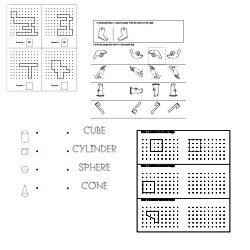First Grade Geometry Printables, Worksheets, and Lessons

Shapes

Matching Polygons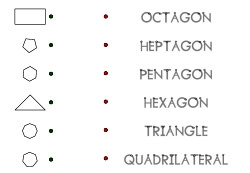Matching Polygons

Shapes - Counting Number of Sides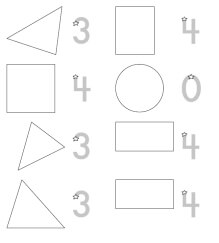Shapes - Counting Number of Sides (traceable answer given for each)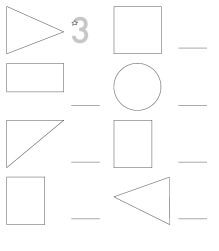Shapes - Counting Number of Sides (traceable answer for first shape only)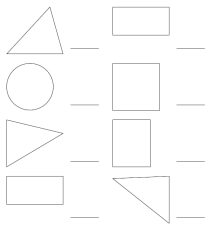Shapes - Counting Number of Sides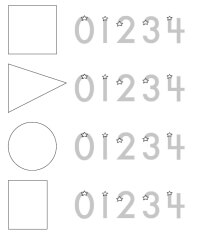Shapes - Counting Number of Sides - Circle the Answer

Draw Shapes and Trace Word - Circle, Rectangle, Square, Triangle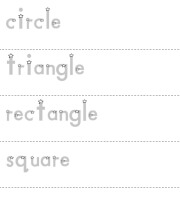Draw Shapes and Trace Word (uppercase words) Draw Shapes and Trace Word (lowercase words)

Complete Shapes - Circle, Rectangle, Square, Triangle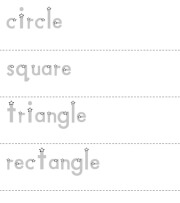Complete the Shapes

First Grade Geometry: Understanding Shape Words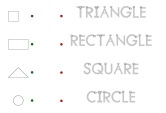Match Shapes with Spelling of Word

Writing Uppercase Letters to the Left and Right
Writing Uppercase Letters to the Left and Right - Easier: Pictorial Directions Given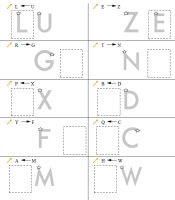Left and Right: Trace Hint On Each - First Two Trace Answers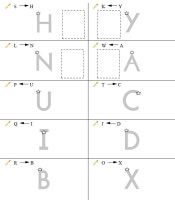Left and Right: Trace Hint On First 4 Problems - First Two Trace Answers Left and Right: Trace Hint On First 4 Problems

Writing Uppercase Letters to the Left and Right - Pictorial And English Directions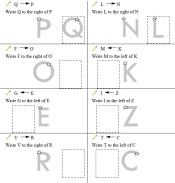Left and Right: Trace Hint On Each - First Two Trace Answers Left and Right: Trace Hint On First 4 Problems - First Two Trace Answers Left and Right: Trace Hint On First 4 Problems

Writing Uppercase Letters to the Left and Right - Pictorial Directions for First Two Problems then English Directions Only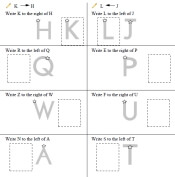Left and Right: Trace Hint On Each - First Two Trace Answers Left and Right: Trace Hint On First 4 Problems - First Two Trace Answers Left and Right: Trace Hint On First 4 Problems

Writing Uppercase Letters to the Left and Right - English Directions Only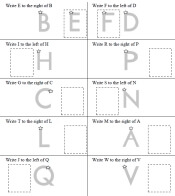Left and Right: Trace Hint On Each - First Two Trace Answers Left and Right: Trace Hint On First 4 Problems - First Two Trace Answers Left and Right: Trace Hint On First 4 Problems

Left and Right, Above and Below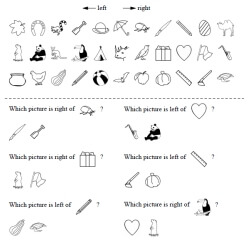Left and Right, Above and Below

Following Directions - Locations: Right, Left, Below, and Above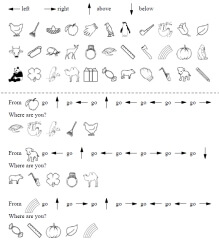Following Directions

Solid Figures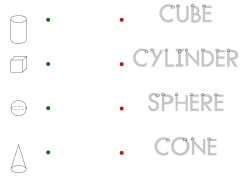Matching Solid Figures with Traceable Words (rectangular prism not included)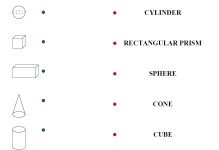Matching Solid Figures with Words

Matching Solid Figures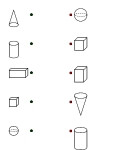Match the Solid Figures

Tracing and then Drawing Solid Figures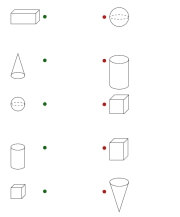Trace and Draw a Cube (larger cubes) Trace and Draw a Cube (smaller cubes)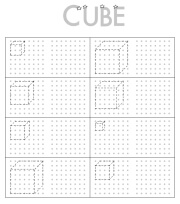Trace and Draw a Rectangular Prism (larger rectangular prisms) Trace and Draw a Rectangular Prism (smaller rectangular prisms)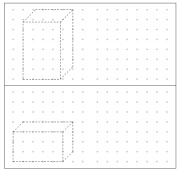Trace and Draw a Cone (larger cones) Trace and Draw a Cone (smaller cones)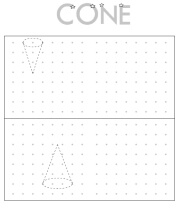Trace and Draw a Cylinder (larger cylinders) Trace and Draw a Cylinder (smaller cylinders)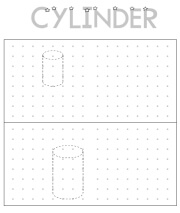Trace and Draw a Sphere (larger spheres) Trace and Draw a Sphere (smaller spheres)

Symmetry and Lines of Symmetry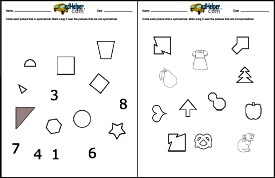Symmetry and Lines of Symmetry

Transformations

Introduction to Horizontal Flips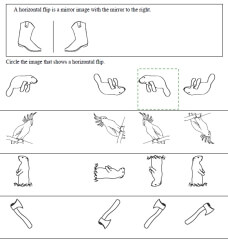Horizontal Flips: Circle the image - first answer given - pictures Horizontal Flips: Circle the image - first answer given - letters and pictures Horizontal Flips: Circle the image - letters and pictures Horizontal Flips: Circle the image - letters and pictures - no instructions with sample at top Horizontal Flips: Circle the image - first answer given - letters

Introduction to Vertical Flips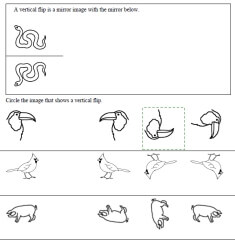Vertical Flips: Circle the image - first answer given - pictures Vertical Flips: Circle the image - first answer given - letters and pictures Vertical Flips: Circle the image - letters and pictures Vertical Flips: Circle the image - letters and pictures - no instructions with sample at top Vertical Flips: Circle the image - first answer given - letters

Introduction to Clockwise Turns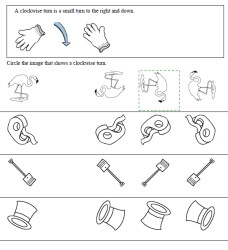Clockwise Turns: Circle the image - first answer given - pictures Clockwise Turns: Circle the image - first answer given - letters and pictures Clockwise Turns: Circle the image - letters and pictures Clockwise Turns: Circle the image - letters and pictures - no instructions with sample at top Clockwise Turns: Circle the image - first answer given - letters

Introduction to Counterclockwise Turns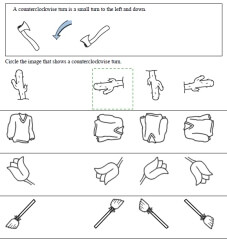Counterclockwise Turns: Circle the image - first answer given - pictures Counterclockwise Turns: Circle the image - first answer given - letters and pictures Counterclockwise Turns: Circle the image - letters and pictures Counterclockwise Turns: Circle the image - letters and pictures - no instructions with sample at top Counterclockwise Turns: Circle the image - first answer given - letters

Drawing Slides, Flips, and Turns
Drawing Slides - Easier (just says to draw a slide, no specific directions)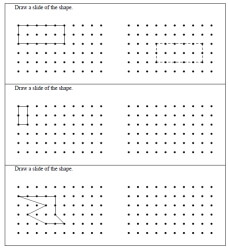Drawing Slides - trace answers given to each Drawing Slides - trace answers given to first problem only Drawing Slides

Drawing Slides - Exact Directions Given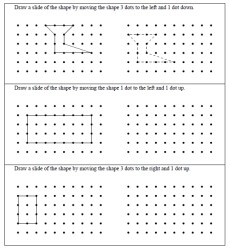Drawing Slides - trace answers given to each Drawing Slides - trace answers given to first problem only Drawing Slides

Drawing Horizontal Flips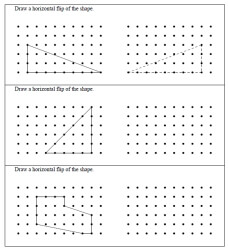Drawing Horizontal Flips - trace answers given to each Drawing Horizontal Flips - trace answers given to first problem only Drawing Horizontal Flips

Drawing Vertical Flips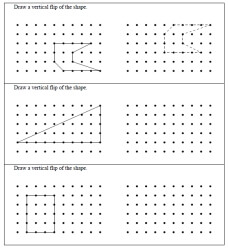Drawing Vertical Flips - trace answers given to each Drawing Vertical Flips - trace answers given to first problem only Drawing Vertical Flips

Drawing Flips - Horizontal and Vertical Flips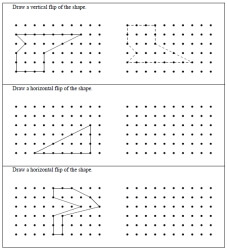Drawing Flips - trace answers given to each Drawing Flips - trace answers given to first problem only Drawing Flips

Drawing Clockwise Turns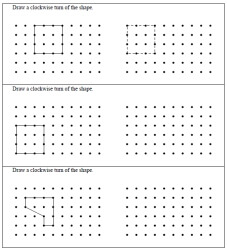Drawing Clockwise Turns - trace answers given to each Drawing Clockwise Turns - trace answers given to first problem only Drawing Clockwise Turns

Drawing Counterclockwise Turns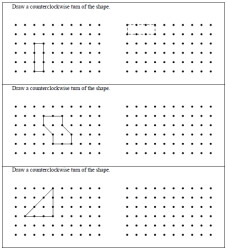Drawing Counterclockwise Turns - trace answers given to each Drawing Counterclockwise Turns - trace answers given to first problem only Drawing Counterclockwise Turns

Drawing Turns - Clockwise and Counterclockwise Turns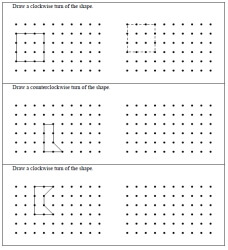Drawing Turns - trace answers given to each Drawing Turns - trace answers given to first problem only Drawing Turns

Drawing Slides, Turns, and Flips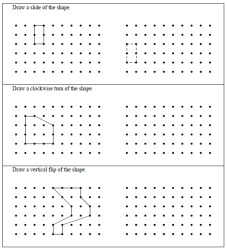Drawing Slides, Turns, and Flips - trace answers given to each Drawing Slides, Turns, and Flips - trace answers given to first problem only Drawing Slides, Turns, and Flips

Flips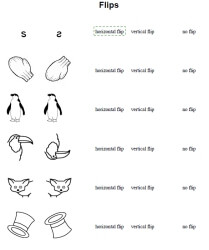Flips - pictures - multiple choice: circle horizontal flip, vertical flip, or no flip Flips - letters or pictures - multiple choice: circle horizontal flip, vertical flip, or no flip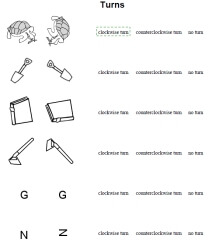Flips - smaller pictures (more problems on a page) - multiple choice: circle horizontal flip, vertical flip, or no flip Flips - smaller letters or smaller pictures (more problems on a page) - multiple choice: circle horizontal flip, vertical flip, or no flip

Turns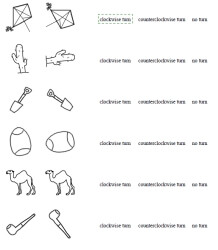Turns - pictures - multiple choice: circle clockwise turn, counterclockwise turn, or no turn Turns - letters or pictures - multiple choice: circle clockwise turn, counterclockwise turn, or no turn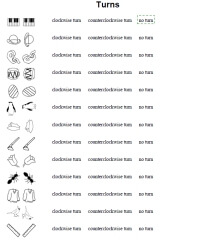Turns - smaller pictures (more problems on a page) - multiple choice: circle clockwise turn, counterclockwise turn, or no turn Turns - smaller letters or smaller pictures (more problems on a page) - multiple choice: circle clockwise turn, counterclockwise turn, or no turn

Flips and Turns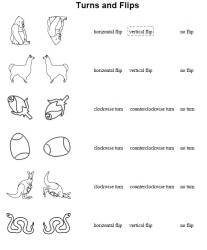Flips and Turns - pictures Flips and Turns - letters or pictures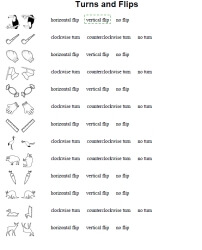Flips and Turns - smaller pictures (more problems on a page) Flips and Turns - smaller letters or smaller pictures (more problems on a page)

Shape Mazes

Ordered Pairs Printables

Perimeter

Have a suggestion or would like to leave feedback?